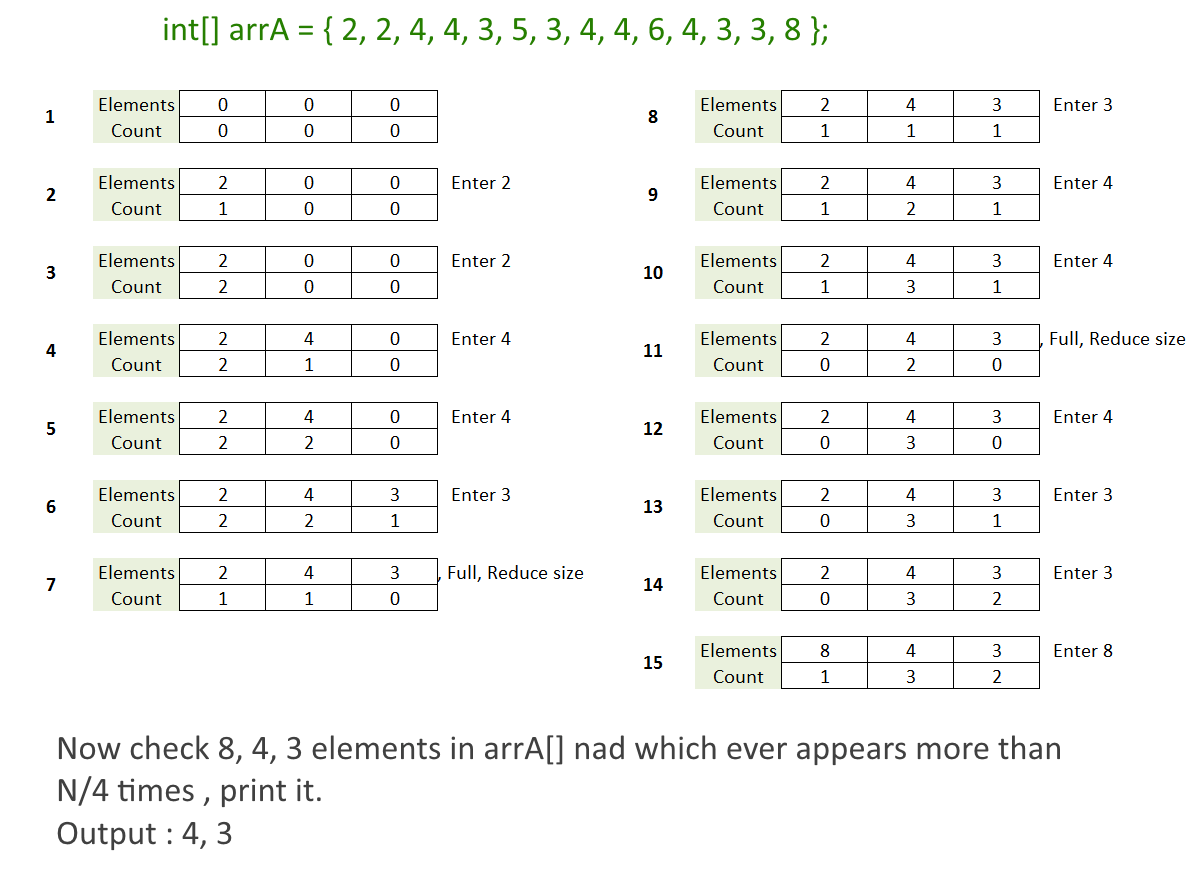Hard

# Find All Elements in an Array which appears more than N/K times, N is Array Size and k is a Number.

Objective: Given an array of size of N and number k. Find all elements in an Array which appear more than N/K times.

Input: Array [] and number k.

Example:

```int[] arrA = { 2, 2, 4, 4, 3, 5, 3, 4, 4, 6, 4, 3, 3, 8 };

K = 4

N/k = 14/4 = 3

Output will be [3,4] they appear 5, 4 times respectively.
```

Approach:

Naive Approach: Take two for loops , check every element with all other elements, Time Complexity -   O(n2) time.

Better Approach: Tetris Game technique- O(Nk)

• We will create a class structure which will store an element and its count.

class

``` Elements{
int element;
int count;
public Elements(
int element,
int count){
this.element = element;
this.count =count;
}
}
```
• Create array etms[] contains k-1 objects of class Elements with element =0 and count = 0.
• So idea is, to navigate all the elements of the given array.
• Check if an element exists in etms[] if not, insert it with the count 1 and if exists then increase its count.
• Also check if etms[] gets full when inserting an element, if it is not, follow the previous step. If it is full then reduce the count of every existing element in the etms[]. (Just think of a Tetris game, when a row gets full, it gets deleted and the size of the Tetris is reduced by 1) see the picture below.
• Once all the elements of the array get over, check every element of etms[] with the array and print those elements which have N/K times.Find All Elements in an Array that appear more than NbyK times

Code:

Output:

```4 appears more than n/4 times, Actual count is 5
3 appears more than n/4 times, Actual count is 4
```